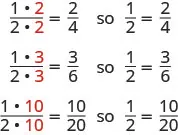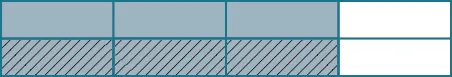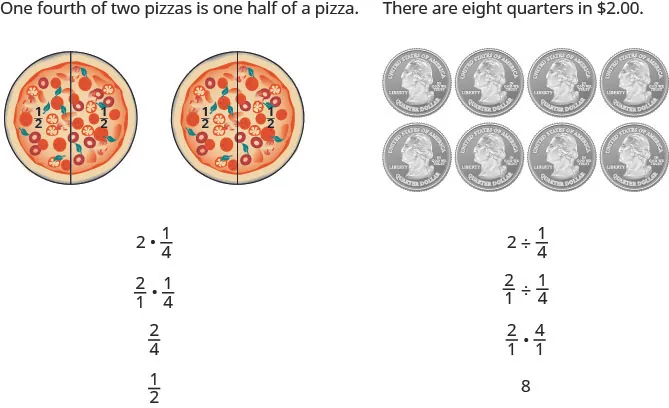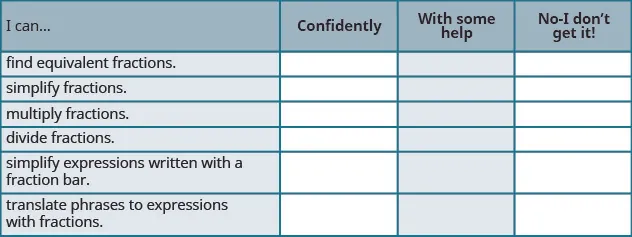Elementary Algebra 2e

# 1.5Visualize Fractions

Elementary Algebra 2e1.5 Visualize Fractions

### Learning Objectives

By the end of this section, you will be able to:

• Find equivalent fractions
• Simplify fractions
• Multiply fractions
• Divide fractions
• Simplify expressions written with a fraction bar
• Translate phrases to expressions with fractions

### Be Prepared 1.5

A more thorough introduction to the topics covered in this section can be found in the Prealgebra chapter, Fractions.

### Find Equivalent Fractions

Fractions are a way to represent parts of a whole. The fraction $1313$ means that one whole has been divided into 3 equal parts and each part is one of the three equal parts. See Figure 1.11. The fraction $2323$ represents two of three equal parts. In the fraction $23,23,$ the 2 is called the numerator and the 3 is called the denominator.

Figure 1.11 The circle on the left has been divided into 3 equal parts. Each part is $1313$ of the 3 equal parts. In the circle on the right, $2323$ of the circle is shaded (2 of the 3 equal parts).

### Manipulative Mathematics

Doing the Manipulative Mathematics activity “Model Fractions” will help you develop a better understanding of fractions, their numerators and denominators.

### Fraction

A fraction is written $ab,ab,$ where $b≠0b≠0$ and

• a is the numerator and b is the denominator.

A fraction represents parts of a whole. The denominator b is the number of equal parts the whole has been divided into, and the numerator a indicates how many parts are included.

If a whole pie has been cut into 6 pieces and we eat all 6 pieces, we ate $6666$ pieces, or, in other words, one whole pie.So $66=1.66=1.$ This leads us to the property of one that tells us that any number, except zero, divided by itself is 1.

### Property of One

$aa=1(a≠0)aa=1(a≠0)$

Any number, except zero, divided by itself is one.

### Manipulative Mathematics

Doing the Manipulative Mathematics activity “Fractions Equivalent to One” will help you develop a better understanding of fractions that are equivalent to one.

If a pie was cut in $66$ pieces and we ate all 6, we ate $6666$ pieces, or, in other words, one whole pie. If the pie was cut into 8 pieces and we ate all 8, we ate $8888$ pieces, or one whole pie. We ate the same amount—one whole pie.

The fractions $6666$ and $8888$ have the same value, 1, and so they are called equivalent fractions. Equivalent fractions are fractions that have the same value.

Let’s think of pizzas this time. Figure 1.12 shows two images: a single pizza on the left, cut into two equal pieces, and a second pizza of the same size, cut into eight pieces on the right. This is a way to show that $1212$ is equivalent to $48.48.$ In other words, they are equivalent fractions.

Figure 1.12 Since the same amount is of each pizza is shaded, we see that $1212$ is equivalent to $48.48.$ They are equivalent fractions.

### Equivalent Fractions

Equivalent fractions are fractions that have the same value.

How can we use mathematics to change $1212$ into $48?48?$ How could we take a pizza that is cut into 2 pieces and cut it into 8 pieces? We could cut each of the 2 larger pieces into 4 smaller pieces! The whole pizza would then be cut into $88$ pieces instead of just 2. Mathematically, what we’ve described could be written like this as $1·42·4=48.1·42·4=48.$ See Figure 1.13.

Figure 1.13 Cutting each half of the pizza into $44$ pieces, gives us pizza cut into 8 pieces: $1·42·4=48.1·42·4=48.$

This model leads to the following property:

### Equivalent Fractions Property

If $a,b,ca,b,c$ are numbers where $b≠0,c≠0,b≠0,c≠0,$ then

$ab=a·cb·cab=a·cb·c$

If we had cut the pizza differently, we could getSo, we say $12,24,36,and102012,24,36,and1020$ are equivalent fractions.

### Manipulative Mathematics

Doing the Manipulative Mathematics activity “Equivalent Fractions” will help you develop a better understanding of what it means when two fractions are equivalent.

### Example 1.64

Find three fractions equivalent to $25.25.$

### Try It 1.127

Find three fractions equivalent to $35.35.$

### Try It 1.128

Find three fractions equivalent to $45.45.$

### Simplify Fractions

A fraction is considered simplified if there are no common factors, other than 1, in its numerator and denominator.

For example,

• $2323$ is simplified because there are no common factors of 2 and 3.
• $10151015$ is not simplified because $55$ is a common factor of 10 and 15.

### Simplified Fraction

A fraction is considered simplified if there are no common factors in its numerator and denominator.

The phrase reduce a fraction means to simplify the fraction. We simplify, or reduce, a fraction by removing the common factors of the numerator and denominator. A fraction is not simplified until all common factors have been removed. If an expression has fractions, it is not completely simplified until the fractions are simplified.

In Example 1.64, we used the equivalent fractions property to find equivalent fractions. Now we’ll use the equivalent fractions property in reverse to simplify fractions. We can rewrite the property to show both forms together.

### Equivalent Fractions Property

If $a,b,ca,b,c$ are numbers where $b≠0,c≠0,b≠0,c≠0,$

$thenab=a·cb·canda·cb·c=abthenab=a·cb·canda·cb·c=ab$

### Example 1.65

Simplify: $−3256.−3256.$

### Try It 1.129

Simplify: $−4254.−4254.$

### Try It 1.130

Simplify: $−4581.−4581.$

Sometimes it may not be easy to find common factors of the numerator and denominator. When this happens, a good idea is to factor the numerator and the denominator into prime numbers. Then divide out the common factors using the equivalent fractions property.

### Example 1.66

#### How to Simplify a Fraction

Simplify: $−210385.−210385.$

### Try It 1.131

Simplify: $−69120.−69120.$

### Try It 1.132

Simplify: $−120192.−120192.$

We now summarize the steps you should follow to simplify fractions.

### How To

#### Simplify a Fraction.

1. Step 1. Rewrite the numerator and denominator to show the common factors.
If needed, factor the numerator and denominator into prime numbers first.
2. Step 2. Simplify using the equivalent fractions property by dividing out common factors.
3. Step 3. Multiply any remaining factors, if needed.

### Example 1.67

Simplify: $5x5y.5x5y.$

### Try It 1.133

Simplify: $7x7y.7x7y.$

### Try It 1.134

Simplify: $3a3b.3a3b.$

### Multiply Fractions

Many people find multiplying and dividing fractions easier than adding and subtracting fractions. So we will start with fraction multiplication.

### Manipulative Mathematics

Doing the Manipulative Mathematics activity “Model Fraction Multiplication” will help you develop a better understanding of multiplying fractions.

We’ll use a model to show you how to multiply two fractions and to help you remember the procedure. Let’s start with $34.34.$Now we’ll take $1212$ of $34.34.$Notice that now, the whole is divided into 8 equal parts. So $12·34=38.12·34=38.$

To multiply fractions, we multiply the numerators and multiply the denominators.

### Fraction Multiplication

If $a,b,candda,b,candd$ are numbers where $b≠0andd≠0,b≠0andd≠0,$ then

$ab·cd=acbdab·cd=acbd$

To multiply fractions, multiply the numerators and multiply the denominators.

When multiplying fractions, the properties of positive and negative numbers still apply, of course. It is a good idea to determine the sign of the product as the first step. In Example 1.68, we will multiply negative and a positive, so the product will be negative.

### Example 1.68

Multiply: $−1112·57.−1112·57.$

### Try It 1.135

Multiply: $−1028·815.−1028·815.$

### Try It 1.136

Multiply: $−920·512.−920·512.$

When multiplying a fraction by an integer, it may be helpful to write the integer as a fraction. Any integer, a, can be written as $a1.a1.$ So, for example, $3=31.3=31.$

### Example 1.69

Multiply: $−125(−20x).−125(−20x).$

### Try It 1.137

Multiply: $113(−9a).113(−9a).$

### Try It 1.138

Multiply: $137(−14b).137(−14b).$

### Divide Fractions

Now that we know how to multiply fractions, we are almost ready to divide. Before we can do that, we need some vocabulary.

The reciprocal of a fraction is found by inverting the fraction, placing the numerator in the denominator and the denominator in the numerator. The reciprocal of $2323$ is $32.32.$

Notice that $23·32=1.23·32=1.$ A number and its reciprocal multiply to 1.

To get a product of positive 1 when multiplying two numbers, the numbers must have the same sign. So reciprocals must have the same sign.

The reciprocal of $−107−107$ is $−710,−710,$ since $−107(−710)=1.−107(−710)=1.$

### Reciprocal

The reciprocal of $abab$ is $ba.ba.$

A number and its reciprocal multiply to one $ab·ba=1.ab·ba=1.$

### Manipulative Mathematics

Doing the Manipulative Mathematics activity “Model Fraction Division” will help you develop a better understanding of dividing fractions.

To divide fractions, we multiply the first fraction by the reciprocal of the second.

### Fraction Division

If $a,b,candda,b,candd$ are numbers where $b≠0,c≠0andd≠0,b≠0,c≠0andd≠0,$ then

$ab÷cd=ab·dcab÷cd=ab·dc$

To divide fractions, we multiply the first fraction by the reciprocal of the second.

We need to say $b≠0,c≠0andd≠0b≠0,c≠0andd≠0$ to be sure we don’t divide by zero!

### Example 1.70

Divide: $−23÷n5.−23÷n5.$

### Try It 1.139

Divide: $−35÷p7.−35÷p7.$

### Try It 1.140

Divide: $−58÷q3.−58÷q3.$

### Example 1.71

Find the quotient: $−718÷(−1427).−718÷(−1427).$

### Try It 1.141

Find the quotient: $−727÷(−3536).−727÷(−3536).$

### Try It 1.142

Find the quotient: $−514÷(−1528).−514÷(−1528).$

There are several ways to remember which steps to take to multiply or divide fractions. One way is to repeat the call outs to yourself. If you do this each time you do an exercise, you will have the steps memorized.

• “To multiply fractions, multiply the numerators and multiply the denominators.”
• “To divide fractions, multiply the first fraction by the reciprocal of the second.”

Another way is to keep two examples in mind:The numerators or denominators of some fractions contain fractions themselves. A fraction in which the numerator or the denominator is a fraction is called a complex fraction.

### Complex Fraction

A complex fraction is a fraction in which the numerator or the denominator contains a fraction.

Some examples of complex fractions are:

$6733458x2566733458x256$

To simplify a complex fraction, we remember that the fraction bar means division. For example, the complex fraction $34583458$ means $34÷58.34÷58.$

### Example 1.72

Simplify: $3458.3458.$

### Try It 1.143

Simplify: $2356.2356.$

### Try It 1.144

Simplify: $37611.37611.$

### Example 1.73

Simplify: $x2xy6.x2xy6.$

### Try It 1.145

Simplify: $a8ab6.a8ab6.$

### Try It 1.146

Simplify: $p2pq8.p2pq8.$

### Simplify Expressions with a Fraction Bar

The line that separates the numerator from the denominator in a fraction is called a fraction bar. A fraction bar acts as grouping symbol. The order of operations then tells us to simplify the numerator and then the denominator. Then we divide.

To simplify the expression $5−37+1,5−37+1,$ we first simplify the numerator and the denominator separately. Then we divide.

$5−37+15−37+1$
$2828$
$1414$

### How To

#### Simplify an Expression with a Fraction Bar.

1. Step 1. Simplify the expression in the numerator. Simplify the expression in the denominator.
2. Step 2. Simplify the fraction.

### Example 1.74

Simplify: $4−2(3)22+2.4−2(3)22+2.$

### Try It 1.147

Simplify: $6−3(5)32+3.6−3(5)32+3.$

### Try It 1.148

Simplify: $4−4(6)32+3.4−4(6)32+3.$

Where does the negative sign go in a fraction? Usually the negative sign is in front of the fraction, but you will sometimes see a fraction with a negative numerator, or sometimes with a negative denominator. Remember that fractions represent division. When the numerator and denominator have different signs, the quotient is negative.

$−13=−13negativepositive=negative1−3=−13positivenegative=negative−13=−13negativepositive=negative1−3=−13positivenegative=negative$

For any positive numbers a and b,

$−ab=a−b=−ab−ab=a−b=−ab$

### Example 1.75

Simplify: $4(−3)+6(−2)−3(2)−2.4(−3)+6(−2)−3(2)−2.$

### Try It 1.149

Simplify: $8(−2)+4(−3)−5(2)+3.8(−2)+4(−3)−5(2)+3.$

### Try It 1.150

Simplify: $7(−1)+9(−3)−5(3)−2.7(−1)+9(−3)−5(3)−2.$

### Translate Phrases to Expressions with Fractions

Now that we have done some work with fractions, we are ready to translate phrases that would result in expressions with fractions.

The English words quotient and ratio are often used to describe fractions. Remember that “quotient” means division. The quotient of $aa$ and $bb$ is the result we get from dividing $aa$ by $b,b,$ or $ab.ab.$

### Example 1.76

Translate the English phrase into an algebraic expression: the quotient of the difference of m and n, and p.

### Try It 1.151

Translate the English phrase into an algebraic expression: the quotient of the difference of a and b, and cd.

### Try It 1.152

Translate the English phrase into an algebraic expression: the quotient of the sum of $pp$ and $q,q,$ and $rr$

### Section 1.5 Exercises

#### Practice Makes Perfect

Find Equivalent Fractions

In the following exercises, find three fractions equivalent to the given fraction. Show your work, using figures or algebra.

343.

$3 8 3 8$

344.

$5 8 5 8$

345.

$5 9 5 9$

346.

$1 8 1 8$

Simplify Fractions

In the following exercises, simplify.

347.

$− 40 88 − 40 88$

348.

$− 63 99 − 63 99$

349.

$− 108 63 − 108 63$

350.

$− 104 48 − 104 48$

351.

$120 252 120 252$

352.

$182 294 182 294$

353.

$− 3 x 12 y − 3 x 12 y$

354.

$− 4 x 32 y − 4 x 32 y$

355.

$14 x 2 21 y 14 x 2 21 y$

356.

$24 a 32 b 2 24 a 32 b 2$

Multiply Fractions

In the following exercises, multiply.

357.

$3 4 · 9 10 3 4 · 9 10$

358.

$4 5 · 2 7 4 5 · 2 7$

359.

$− 2 3 ( − 3 8 ) − 2 3 ( − 3 8 )$

360.

$− 3 4 ( − 4 9 ) − 3 4 ( − 4 9 )$

361.

$− 5 9 · 3 10 − 5 9 · 3 10$

362.

$− 3 8 · 4 15 − 3 8 · 4 15$

363.

$( − 14 15 ) ( 9 20 ) ( − 14 15 ) ( 9 20 )$

364.

$( − 9 10 ) ( 25 33 ) ( − 9 10 ) ( 25 33 )$

365.

$( − 63 84 ) ( − 44 90 ) ( − 63 84 ) ( − 44 90 )$

366.

$( − 33 60 ) ( − 40 88 ) ( − 33 60 ) ( − 40 88 )$

367.

$4 · 5 11 4 · 5 11$

368.

$5 · 8 3 5 · 8 3$

369.

$3 7 · 21 n 3 7 · 21 n$

370.

$5 6 · 30 m 5 6 · 30 m$

371.

$−8 ( 17 4 ) −8 ( 17 4 )$

372.

$( −1 ) ( − 6 7 ) ( −1 ) ( − 6 7 )$

Divide Fractions

In the following exercises, divide.

373.

$3 4 ÷ 2 3 3 4 ÷ 2 3$

374.

$4 5 ÷ 3 4 4 5 ÷ 3 4$

375.

$− 7 9 ÷ ( − 7 4 ) − 7 9 ÷ ( − 7 4 )$

376.

$− 5 6 ÷ ( − 5 6 ) − 5 6 ÷ ( − 5 6 )$

377.

$3 4 ÷ x 11 3 4 ÷ x 11$

378.

$2 5 ÷ y 9 2 5 ÷ y 9$

379.

$5 18 ÷ ( − 15 24 ) 5 18 ÷ ( − 15 24 )$

380.

$7 18 ÷ ( − 14 27 ) 7 18 ÷ ( − 14 27 )$

381.

$8 u 15 ÷ 12 v 25 8 u 15 ÷ 12 v 25$

382.

$12 r 25 ÷ 18 s 35 12 r 25 ÷ 18 s 35$

383.

$−5 ÷ 1 2 −5 ÷ 1 2$

384.

$−3 ÷ 1 4 −3 ÷ 1 4$

385.

$3 4 ÷ ( −12 ) 3 4 ÷ ( −12 )$

386.

$−15 ÷ ( − 5 3 ) −15 ÷ ( − 5 3 )$

In the following exercises, simplify.

387.

$− 8 21 12 35 − 8 21 12 35$

388.

$− 9 16 33 40 − 9 16 33 40$

389.

$− 4 5 2 − 4 5 2$

390.

$5 3 10 5 3 10$

391.

$m 3 n 2 m 3 n 2$

392.

$− 3 8 − y 12 − 3 8 − y 12$

Simplify Expressions Written with a Fraction Bar

In the following exercises, simplify.

393.

$22 + 3 10 22 + 3 10$

394.

$19 − 4 6 19 − 4 6$

395.

$48 24 − 15 48 24 − 15$

396.

$46 4 + 4 46 4 + 4$

397.

$−6 + 6 8 + 4 −6 + 6 8 + 4$

398.

$−6 + 3 17 − 8 −6 + 3 17 − 8$

399.

$4 · 3 6 · 6 4 · 3 6 · 6$

400.

$6 · 6 9 · 2 6 · 6 9 · 2$

401.

$4 2 − 1 25 4 2 − 1 25$

402.

$7 2 + 1 60 7 2 + 1 60$

403.

$8 · 3 + 2 · 9 14 + 3 8 · 3 + 2 · 9 14 + 3$

404.

$9 · 6 − 4 · 7 22 + 3 9 · 6 − 4 · 7 22 + 3$

405.

$5 · 6 − 3 · 4 4 · 5 − 2 · 3 5 · 6 − 3 · 4 4 · 5 − 2 · 3$

406.

$8 · 9 − 7 · 6 5 · 6 − 9 · 2 8 · 9 − 7 · 6 5 · 6 − 9 · 2$

407.

$5 2 − 3 2 3 − 5 5 2 − 3 2 3 − 5$

408.

$6 2 − 4 2 4 − 6 6 2 − 4 2 4 − 6$

409.

$7 · 4 − 2 ( 8 − 5 ) 9 · 3 − 3 · 5 7 · 4 − 2 ( 8 − 5 ) 9 · 3 − 3 · 5$

410.

$9 · 7 − 3 ( 12 − 8 ) 8 · 7 − 6 · 6 9 · 7 − 3 ( 12 − 8 ) 8 · 7 − 6 · 6$

411.

$9 ( 8 − 2 ) − 3 ( 15 − 7 ) 6 ( 7 − 1 ) − 3 ( 17 − 9 ) 9 ( 8 − 2 ) − 3 ( 15 − 7 ) 6 ( 7 − 1 ) − 3 ( 17 − 9 )$

412.

$8 ( 9 − 2 ) − 4 ( 14 − 9 ) 7 ( 8 − 3 ) − 3 ( 16 − 9 ) 8 ( 9 − 2 ) − 4 ( 14 − 9 ) 7 ( 8 − 3 ) − 3 ( 16 − 9 )$

Translate Phrases to Expressions with Fractions

In the following exercises, translate each English phrase into an algebraic expression.

413.

the quotient of r and the sum of s and 10

414.

the quotient of A and the difference of 3 and B

415.

the quotient of the difference of $xandy,and−3xandy,and−3$

416.

the quotient of the sum of $mandn,and4qmandn,and4q$

#### Everyday Math

417.

Baking. A recipe for chocolate chip cookies calls for $3434$ cup brown sugar. Imelda wants to double the recipe. How much brown sugar will Imelda need? Show your calculation. Measuring cups usually come in sets of $14,13,12,and114,13,12,and1$ cup. Draw a diagram to show two different ways that Imelda could measure the brown sugar needed to double the cookie recipe.

418.

Baking. Nina is making 4 pans of fudge to serve after a music recital. For each pan, she needs $2323$ cup of condensed milk. How much condensed milk will Nina need? Show your calculation. Measuring cups usually come in sets of $14,13,12,and114,13,12,and1$ cup. Draw a diagram to show two different ways that Nina could measure the condensed milk needed for $44$ pans of fudge.

419.

Portions Don purchased a bulk package of candy that weighs $55$ pounds. He wants to sell the candy in little bags that hold $1414$ pound. How many little bags of candy can he fill from the bulk package?

420.

Portions Kristen has $3434$ yards of ribbon that she wants to cut into $66$ equal parts to make hair ribbons for her daughter’s 6 dolls. How long will each doll’s hair ribbon be?

#### Writing Exercises

421.

Rafael wanted to order half a medium pizza at a restaurant. The waiter told him that a medium pizza could be cut into 6 or 8 slices. Would he prefer 3 out of 6 slices or 4 out of 8 slices? Rafael replied that since he wasn’t very hungry, he would prefer 3 out of 6 slices. Explain what is wrong with Rafael’s reasoning.

422.

Give an example from everyday life that demonstrates how $12·23is13.12·23is13.$

423.

Explain how you find the reciprocal of a fraction.

424.

Explain how you find the reciprocal of a negative number.

#### Self Check

After completing the exercises, use this checklist to evaluate your mastery of the objectives of this section.After looking at the checklist, do you think you are well prepared for the next section? Why or why not?

Order a print copy

As an Amazon Associate we earn from qualifying purchases.